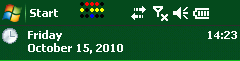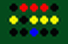Posts tagged ‘today-clock-size-windows-mobile’

## Mobile Development: A binary clock for the taskbar

Hello

do you also like the binary watches from IO?

OK, I like them and was inspired to make some binary clock for the Windows Mobile taskbar.The time shown here is 14:23 displayed as binary dots. The top row (red) shows the hours in 24h format. The second row shows the minutes and the bottom row shows the seconds.What you see here is encoded in binary:```01110   is equal to 0*16 1*8 1*4 1*2 0*1 = 8 + 4 + 2 = 14
10111   is equal to 1*16 0*8 1*4 1*2 1*1 = 16 + 4 + 2 + 1 = 23
00100   is equal to 0*16 0*8 1*4 0*2 0*1 = 4 = 4
```

So you see, it shows the time is 14:23:04